• 利用 Matlab 提供的 MatchedFilter 对象实现了对输入线性FM信号的匹配滤波
• 匹配滤波matlab仿真代码 对于了解匹配滤波原理有用
• 匹配滤波matlab仿真代码 对于了解匹配滤波原理很有用
• 运用matlab实现匹配滤波，文件中有匹配滤波的文档说明
• 匹配滤波源码，详情见http://blog.csdn.net/u013288466/article/details/66473284
• Matched Filtering to Improve SNR for Target DetectionThis example shows how to improve the SNR by performing matched filtering.Place an isotropic antenna element at the global origin (0;...

Matched Filtering to Improve SNR for Target Detection
This example shows how to improve the SNR by performing matched filtering.
Place an isotropic antenna element at the global origin (0;0;0). Then, place a target with a nonfluctuating RCS of 1 square meter approximately 7 km from the transmitter at (5000;5000;10). Set the operating (carrier) frequency to 10 GHz. To simulate a monostatic radar, set the InUseOutputPort property on the transmitter to true. Calculate the range and angle from the transmitter to the target.
antenna = phased.IsotropicAntennaElement('FrequencyRange',[5e9 15e9]);
transmitter = phased.Transmitter('Gain',20,'InUseOutputPort',true);
fc = 10e9;
'MeanRCS',1,'OperatingFrequency',fc);
txloc = [0;0;0];
tgtloc = [5000;5000;10];
transmitterplatform = phased.Platform('InitialPosition',txloc);
targetplatform = phased.Platform('InitialPosition',tgtloc);
[tgtrng,tgtang] = rangeangle(targetplatform.InitialPosition,...
transmitterplatform.InitialPosition);
Create a rectangular pulse waveform 25 μs in duration with a PRF of 10 kHz. Use a single pulse for this example. Determine the maximum unambiguous range for the given PRF. Use the radar equation to determine the peak power required to detect a target. This target has an RCS of 1 square meter at the maximum unambiguous range for the transmitter operating frequency and gain. The SNR is based on a desired false-alarm rate of 1e-6 for a noncoherent detector.
waveform = phased.RectangularWaveform('PulseWidth',25e-6,...
'OutputFormat','Pulses','PRF',10e3,'NumPulses',1);
c = physconst('LightSpeed');
maxrange = c/(2*waveform.PRF);
SNR = npwgnthresh(1e-6,1,'noncoherent');
lambda = physconst('LightSpeed')/target.OperatingFrequency;
Ts = 290;
dbterms = db2pow(SNR - 2*transmitter.Gain);
Pt = (4*pi)^3*physconst('Boltzmann')*Ts/waveform.PulseWidth/target.MeanRCS/(lambda^2)*maxrange^4*dbterms;
Set the peak transmit power to the output value from the radar equation.
transmitter.PeakPower = Pt;
Create radiator and collector objects that operate at 10 GHz. Create a free space path for the propagation of the pulse to and from the target. Then, create a receiver and a matched filter for the rectangular waveform.
'OperatingFrequency',fc,'Sensor',antenna);
channel = phased.FreeSpace('PropagationSpeed',c,...
'OperatingFrequency',fc,'TwoWayPropagation',false);
collector = phased.Collector('PropagationSpeed',c,...
'OperatingFrequency',fc,'Sensor',antenna);
'EnableInputPort',true,'SeedSource','Property','Seed',2e3);
filter = phased.MatchedFilter(...
'Coefficients',getMatchedFilter(waveform),...
'GainOutputPort',true);
After you create all the objects that define your model, you can propagate the pulse to and from the target. Collect the echo at the receiver, and implement the matched filter to improve the SNR.
Generate waveform.
wf = waveform();
Transmit waveform.
[wf,txstatus] = transmitter(wf);
Propagate pulse toward the target.
wf = channel(wf,txloc,tgtloc,[0;0;0],[0;0;0]);
Reflect it off the target.
wf = target(wf);
Propagate the pulse back to transmitter.
wf = channel(wf,tgtloc,txloc,[0;0;0],[0;0;0]);
Collect the echo.
wf = collector(wf,tgtang);
[mf_puls,mfgain] = filter(rx_puls);
Get group delay of matched filter.
Gd = length(filter.Coefficients)-1;
The group delay is constant.
Shift the matched filter output.
mf_puls=[mf_puls(Gd+1:end); mf_puls(1:Gd)];
subplot(2,1,1)
t = unigrid(0,1e-6,1e-4,'[)');
rangegates = c.*t;
rangegates = rangegates/2;
plot(rangegates,abs(rx_puls))
ylabel('Amplitude')
hold on
plot([tgtrng, tgtrng], [0 max(abs(rx_puls))],'r')
subplot(2,1,2)
plot(rangegates,abs(mf_puls))
title('With Matched Filtering')
xlabel('Meters')
ylabel('Amplitude')
hold on
plot([tgtrng, tgtrng], [0 max(abs(mf_puls))],'r')
hold off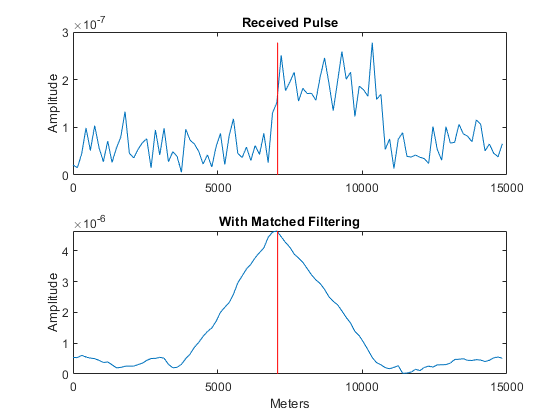展开全文• %%用与发送端相同的根升余弦匹配滤波 yt=y2((size(yf)+1)/2:8:end);%%匹配滤波后抽取 figure(2); stem(x(1:40)); grid;title('原始信号输出'); figure(3); plot(y1(1:100));grid;title('成型信号输出'); figure(4...

转载自：https://blog.csdn.net/yuan1164345228/article/details/45919315

Fd=1;
Fs=8;
Delay=3;
R=0.5;
[yf,tf]=rcosine(Fd,Fs,'fir/sqrt',R,Delay);
figure(1);
plot(yf); grid;title('根升余弦滤波器时域波形')
x=randint(100,1)*2-1;%原始输入信号为+1，-1码
xt=zeros(1,800);
xt(1:8:end)=x; %%插值后信号
y1=filter(yf,tf,xt); %%成型滤波
y2=filter(yf,tf,y1); %%用与发送端相同的根升余弦匹配滤波
yt=y2((size(yf)+1)/2:8:end);%%匹配滤波后抽取
figure(2);
stem(x(1:40)); grid;title('原始信号输出');
figure(3);
plot(y1(1:100));grid;title('成型信号输出');
figure(4);
plot(y2(1:100));grid;title('匹配滤波信号输出');
figure(5);
stem(yt(1:40)); grid;title('抽取后信号输出') ;

滤波器的截止频率是Fd/2；Fs/Fd是内插倍数；其频响可以由滚降系数和窗长(FIR阶数)确定。 delay * (Fs / Fd) * 2 + 1就是滤波器的阶数

转载于:https://www.cnblogs.com/waimen/p/9774730.html
展开全文• 线性调频信号的仿真，及匹配滤波MATLAB代码
• matlab文件以LFM信号为例详细介绍了信号匹配滤波的仿真方法和实现，包括时域方法和频域方法
• matlab实现线性调频信号LFM的匹配滤波算法
• LFM回波信号进行匹配滤波，在雷达数字处理中实现宽发窄收
• 快速实现GFDM和C-FBMC调制/解调，分别包括时域和频域的迫零（ZF）和匹配滤波（MF）均衡； 为OFDM信道估计中使用的压缩感测方法计算字典的功能； 增加信道效应，施加宽带多普勒失真的功能； 通过线性调频Z变换（CZT）...
• 文章目录前言二、仿真1、生成2PAM信号：2、模拟信号的匹配滤波仿真3、LFP的仿真： 前言 二、仿真 1、生成2PAM信号：  理论基础部分： 双极性NRZ矩形脉冲信号的最小平均误码率表现比较优秀，因此选用双极性NRZ...


文章目录
前言一、理论部分二、仿真1、生成2PAM信号2、模拟信号的匹配滤波仿真3、LFP的仿真4、MF的仿真
三、拓展知识1、“假”的[1,1,1,0,1,0,0]序列

前言

一、理论部分
（在书本中，以后补充）
二、仿真
1、生成2PAM信号
理论基础部分：
双极性NRZ矩形脉冲信号的最小平均误码率表现比较优秀，因此选用双极性NRZ矩形脉冲信号作为生成仿真的PAM脉冲信号；matlab中，有一个生成阶跃信号的函数——stepfun(t,t0)，而矩形的脉冲信号就是由一个一个时间长度为1的矩形信号在各个时段内“拼接” 形成。
核心代码部分：
%源信号
t=0:0.01:10;
st_yuan=[1,1,1,0,1,0,0];
st_length=length(st_yuan);
st=0;
st_abs = 1;
for i=1:st_length
if st_yuan(i)==1
st=st_abs*(stepfun(t,i-1)-stepfun(t,i))+st;
elseif st_yuan(i)==0
st=-st_abs*(stepfun(t,i-1)-stepfun(t,i))+st;
end
end

仿真效果展示：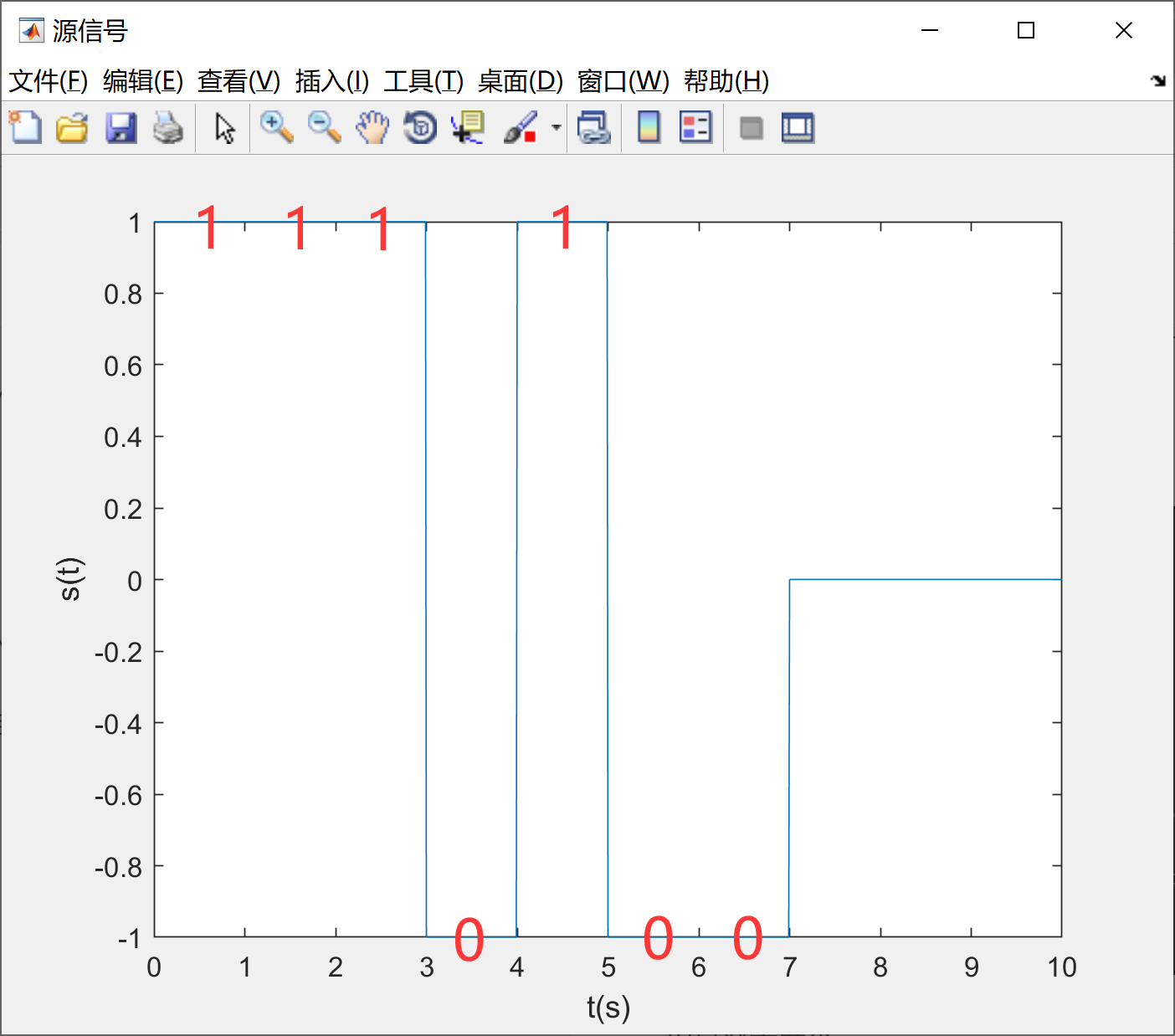2、模拟信号的匹配滤波仿真
理论基础部分：
匹配滤波器的内部，存在一个跟原信号相匹配（时域取反移动）的信号，这个信号和输入信号卷积之后，可以确保在每个周期的最后时刻得到信噪比达到最大；卷积就是能量的累积，而当两个信号完全重合的时候，能量最强，相应地，也就能够确定信号的周期。
核心代码部分：
nt=randn(1,1001);
t=0:0.1:100;
st=0.1*t.*(stepfun(t,0)-stepfun(t,60));
xt=nt+st;
mf=0.1*(60-t).*(stepfun(t,0)-stepfun(t,60));
yt=conv(st,mf);

仿真效果展示：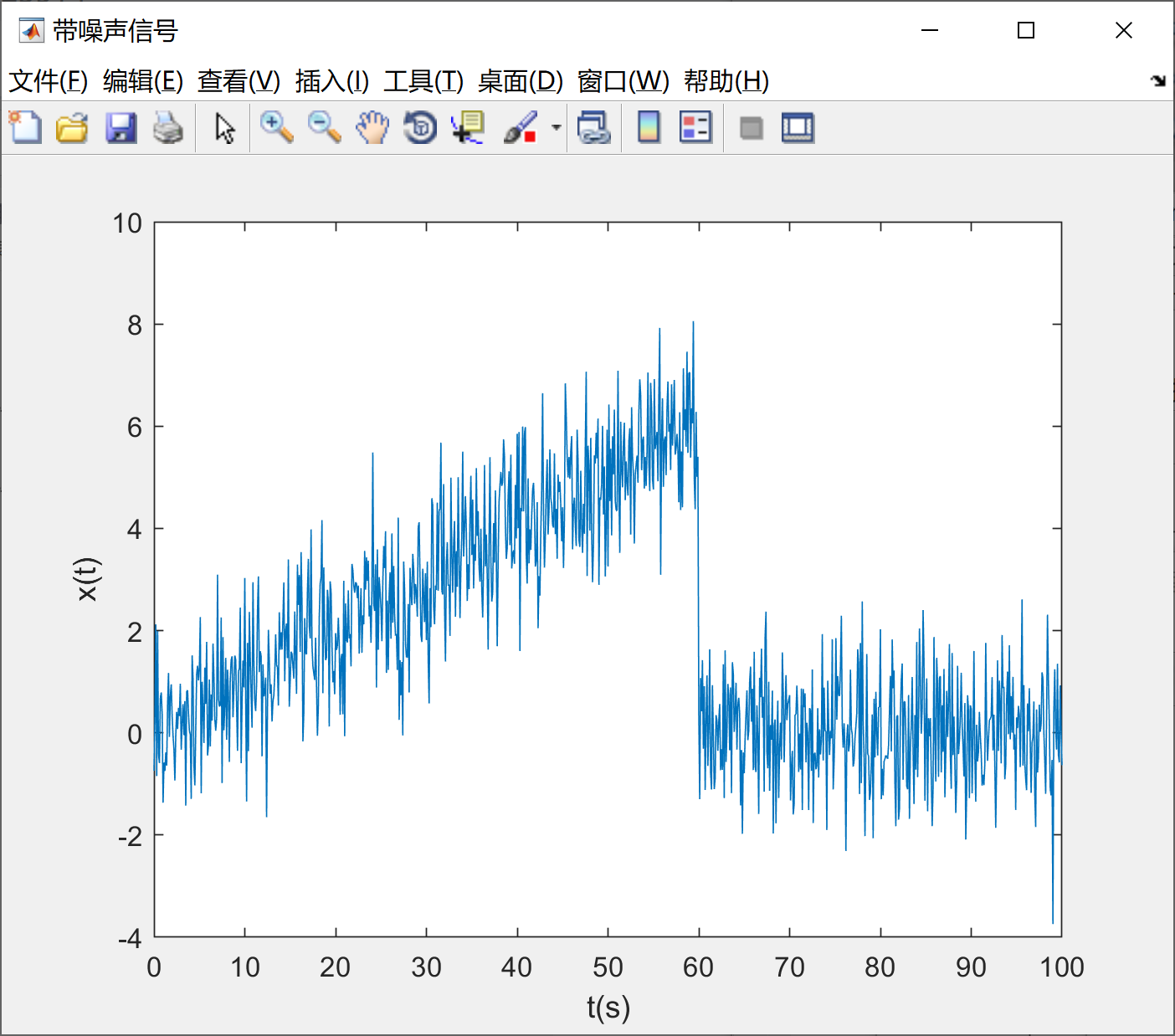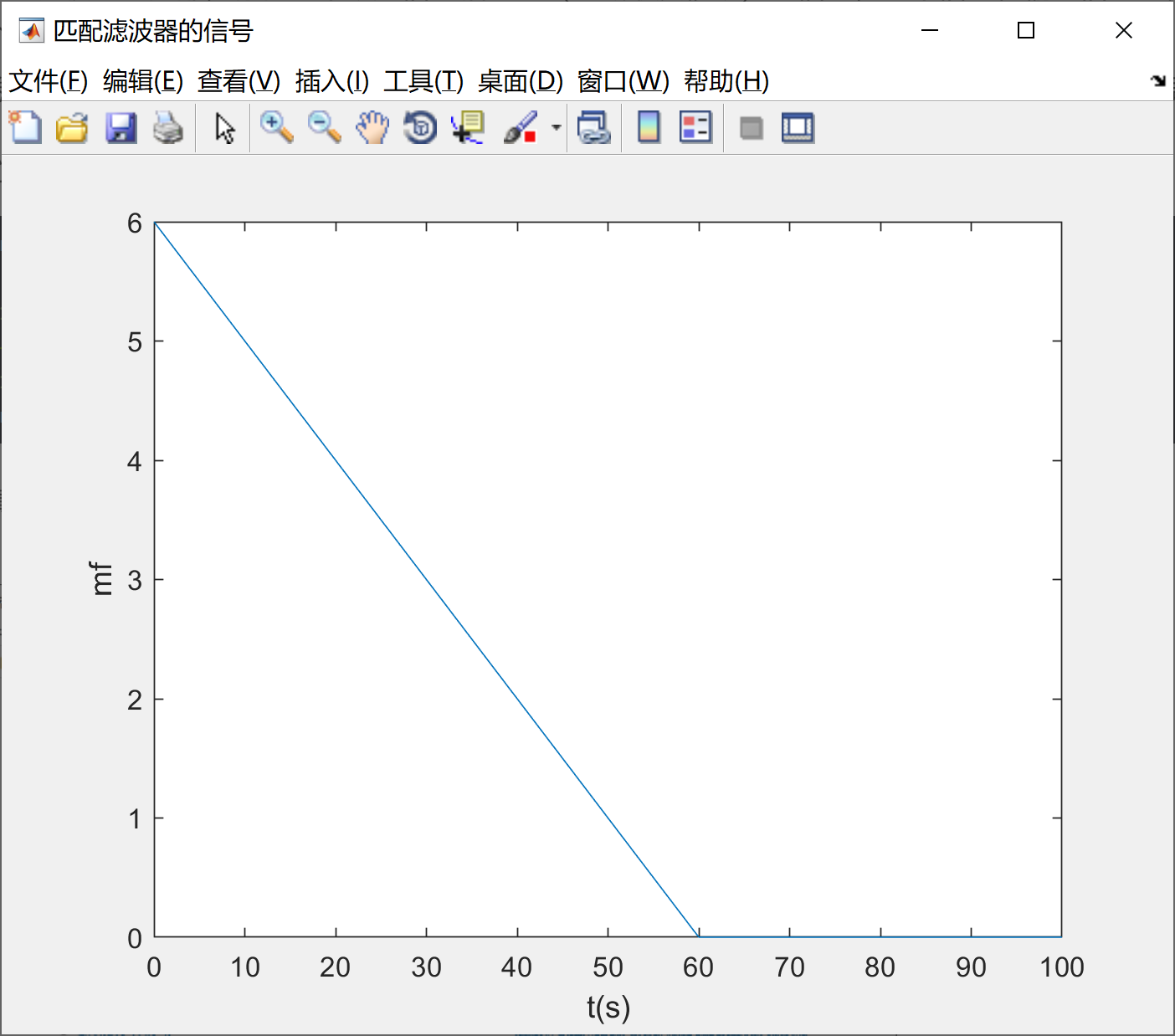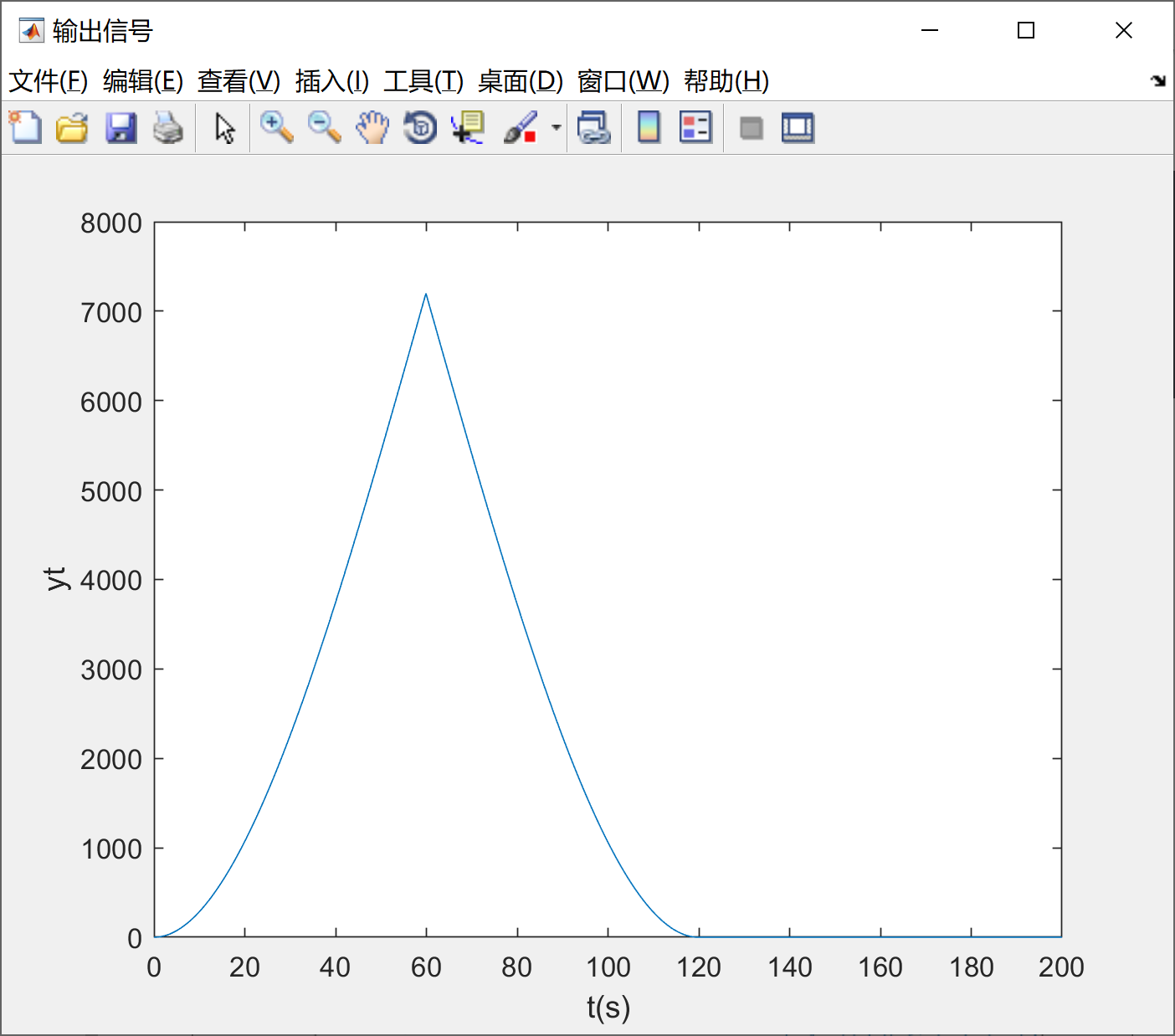3、LFP的仿真
理论基础部分：
由生成源信号的stepfun函数可知，信号的BT=100Hz，而不是BT=1Hz；理论上，低通滤波器的滤波范围应该为100Hz，也就是保证信号的BT都在滤波的范围内，但是为了确保仿真的效果，应该选用B=200Hz。
核心代码部分：
%源信号
t=0:0.01:10;
st_yuan=[1,1,1,0,1,0,0];
st_length=length(st_yuan);
st=0;
for i=1:st_length
if st_yuan(i)==1
st=st_abs*(stepfun(t,i-1)-stepfun(t,i))+st;
elseif st_yuan(i)==0
st=-st_abs*(stepfun(t,i-1)-stepfun(t,i))+st;
end
end
figure('NumberTitle', 'off', 'Name', '源信号');
plot(t,st);
xlabel('t(s)');
ylabel('s(t)');

%信道噪声
nt=randn(1,1001);

%输入信号
xt=st+nt;

%输入信号的FT变换
fs=1000;
xt_length=length(xt);
NFFT_xt = 2^nextpow2(xt_length);
F_XT_domain = (fs/2)*linspace(0,1,NFFT_xt/2);
XT = fft(xt,NFFT_xt);
Amp_XT = 2*abs(XT(1:NFFT_xt/2));

%LPF滤波器
f_LPF=0:1:1023;
LPF=stepfun(f_LPF,0)-stepfun(f_LPF,200);

%滤波
YT=XT.*LPF;

仿真效果展示：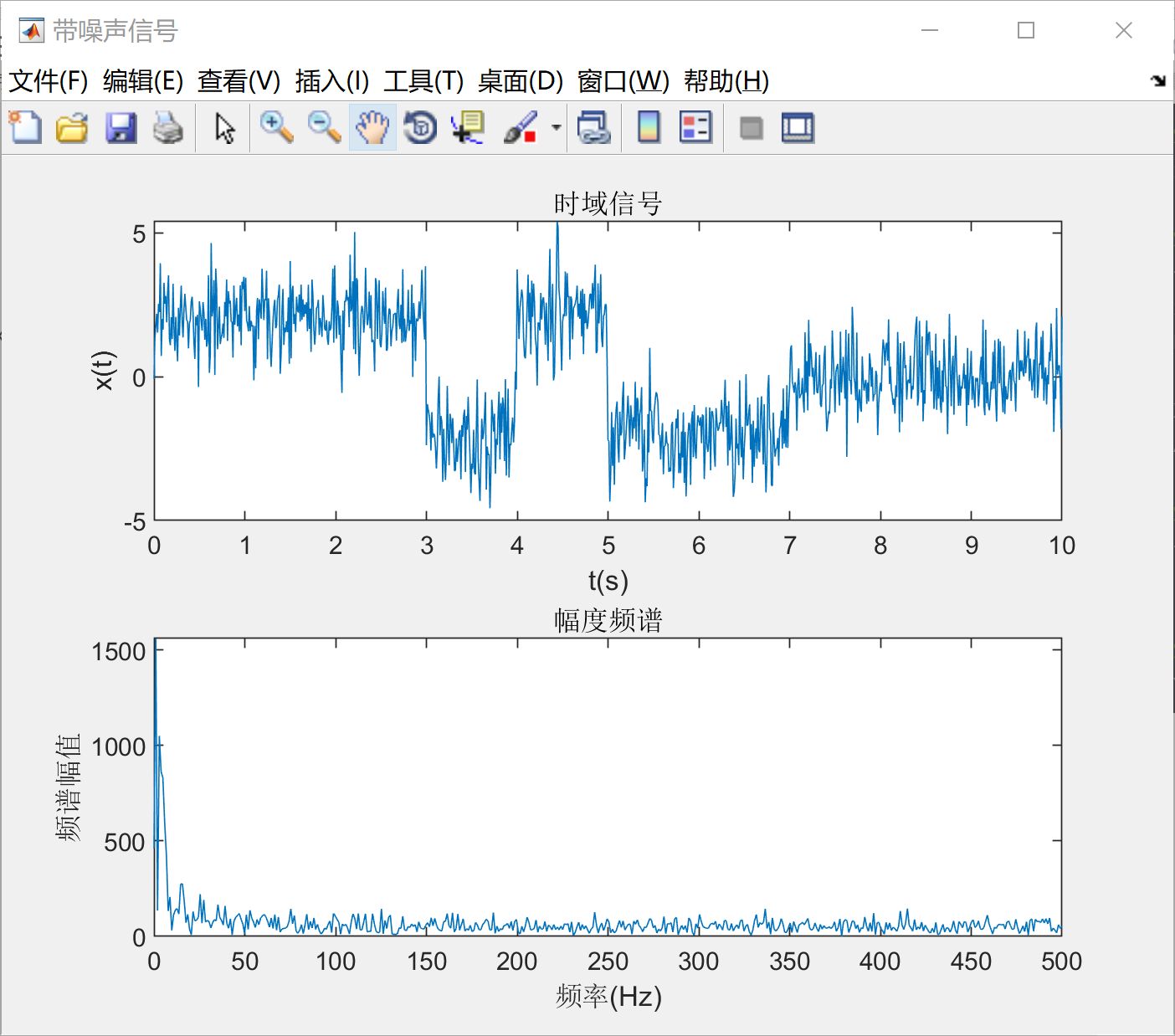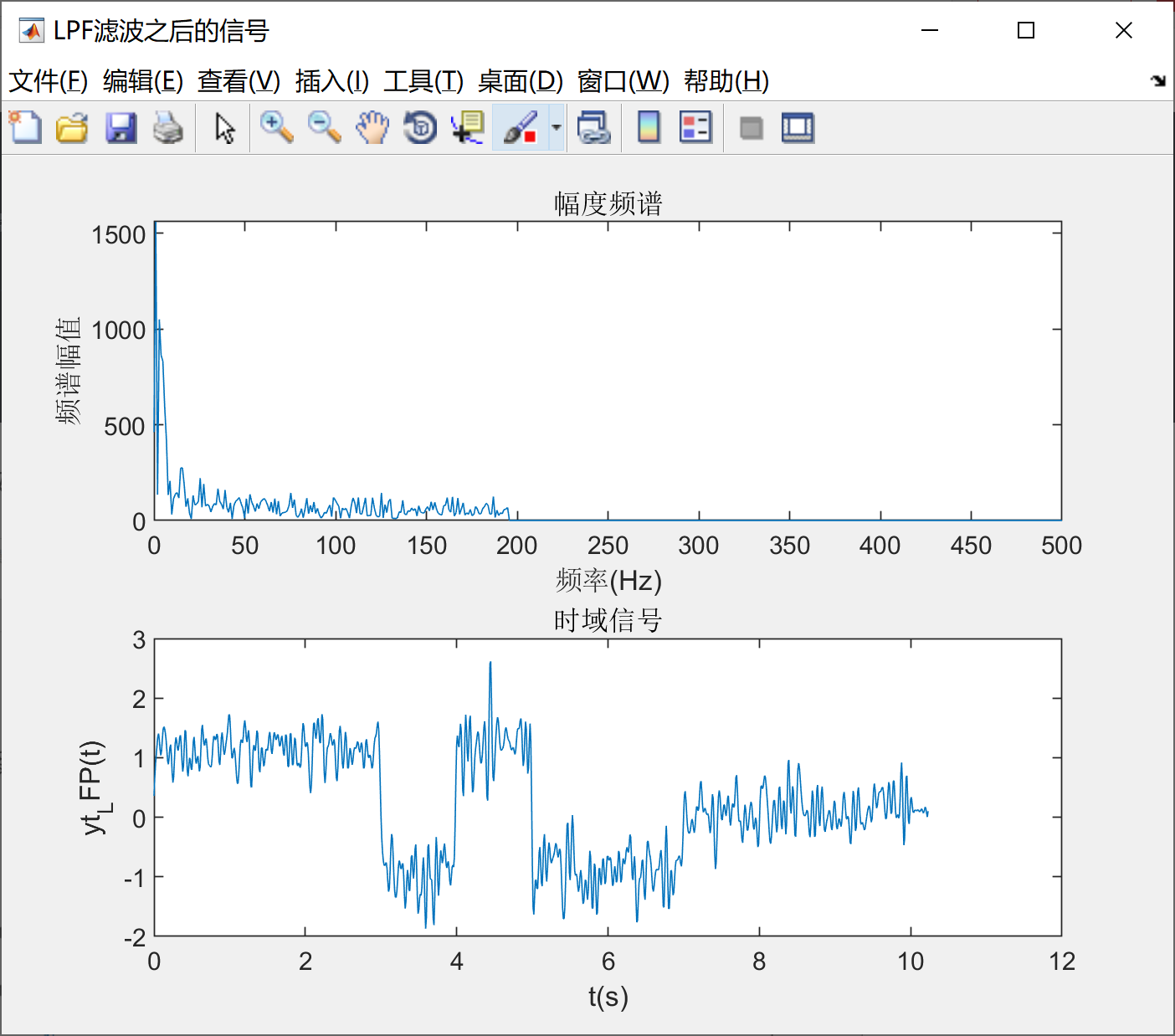4、MF的仿真
理论基础部分：
由匹配滤波的定义可知：MF滤波器里面的匹配信号就是源信号经过时域取反然后移位得到的；MF中的匹配信号是与矩形脉冲信号相匹配的信号，而不是与源信号相匹配的信号；
核心代码部分：
mf=stepfun(t,0)-stepfun(t,1);
yt_MF=conv(xt,mf);
%查看每个周期最后一个时刻的输出信号
sample_yt_MF = [yt_MF(1*100),yt_MF(2*100),yt_MF(3*100),yt_MF(4*100),yt_MF(5*100),yt_MF(6*100),yt_MF(7*100)]

仿真效果展示：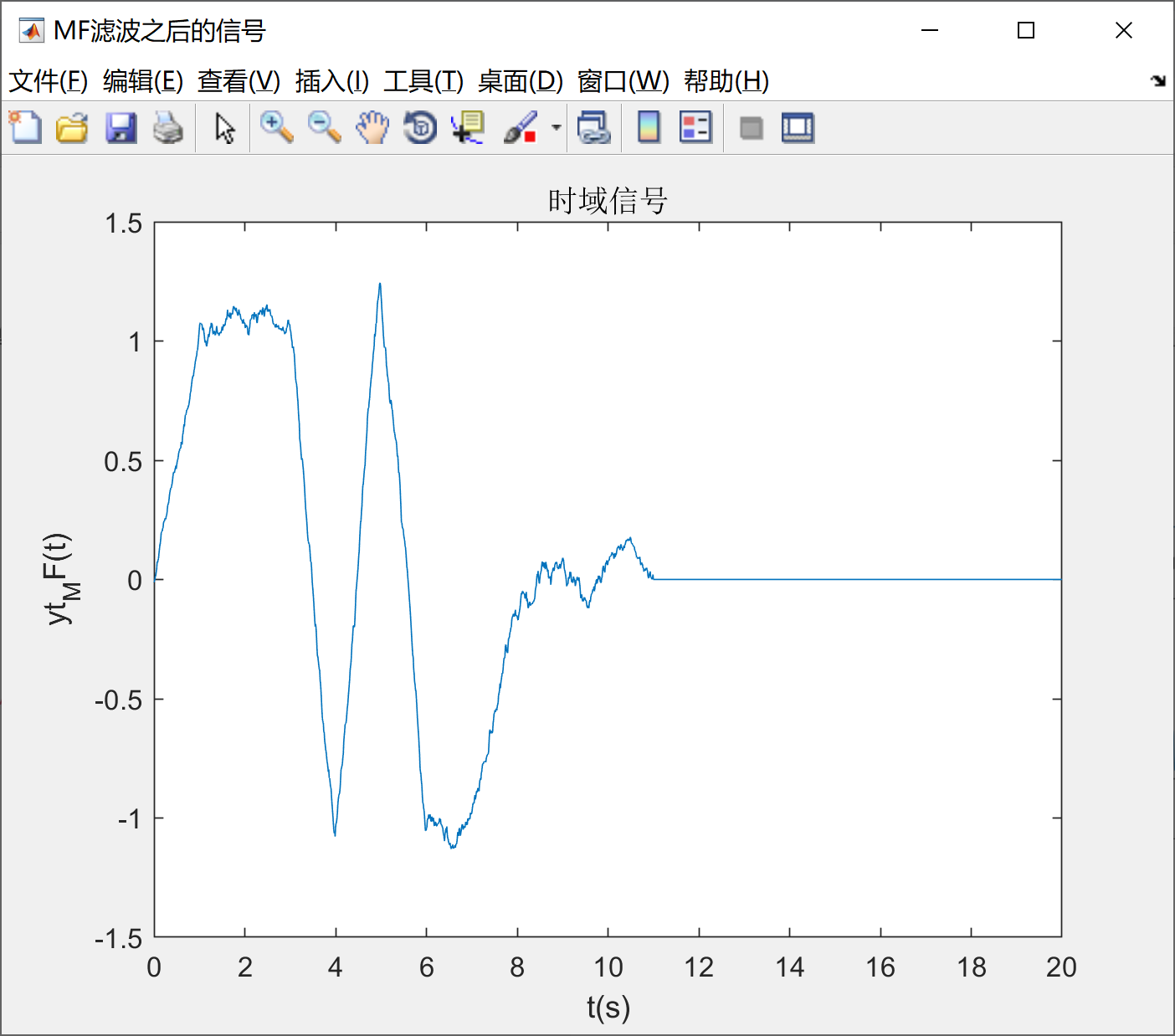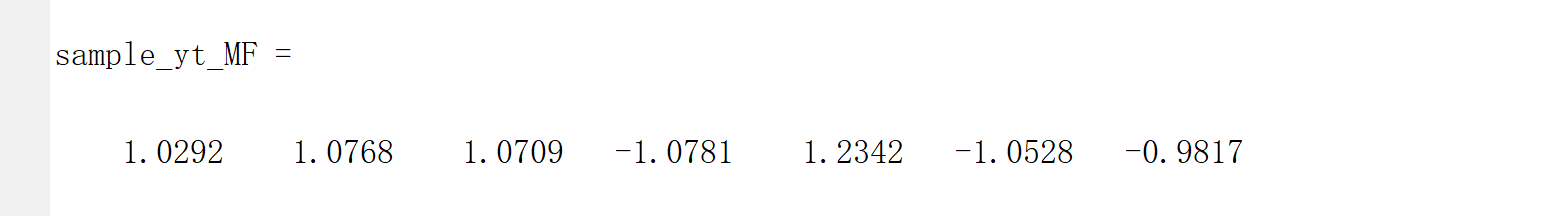三、拓展知识
1、“假”的[1,1,1,0,1,0,0]序列
理论基础：
使用matlab跑信号仿真的时候，我们需要明确一个最基本的概念：MATLAB只能处理或者生成离散的数字序列；在本次仿真中，矩形脉冲信号是使用stepfun() 函数生成的，stepfun(）函数的第一个参数是向量，意思是说，这个参量确定了每两个相邻冲激之间的步进，而这个步进就表明，在时域图中，连续的信号并不连续，只是因为使用了plot(）函数，使得信号看起来是连续的；LPF的滤波带宽参数设置为大于等于两倍源信号的带宽，就可以实现比较好的滤波效果；验证方法：分别使用带宽为B=2Hz和B=200Hz的LPF进行滤波处理，看最后出来的效果图。如果2Hz的LPF得到的输出信号比较平滑，而200Hz的LPF得到的输出信号比较陡峭，那就说明输出信号的带宽为100Hz。
效果验证：
输入信号
%输入信号
fs=1000;
xt_length=length(xt);
NFFT_xt = 2^nextpow2(xt_length);
F_XT_domain = (fs/2)*linspace(0,1,NFFT_xt/2);
XT = fft(xt,NFFT_xt);
Amp_XT = 2*abs(XT(1:NFFT_xt/2));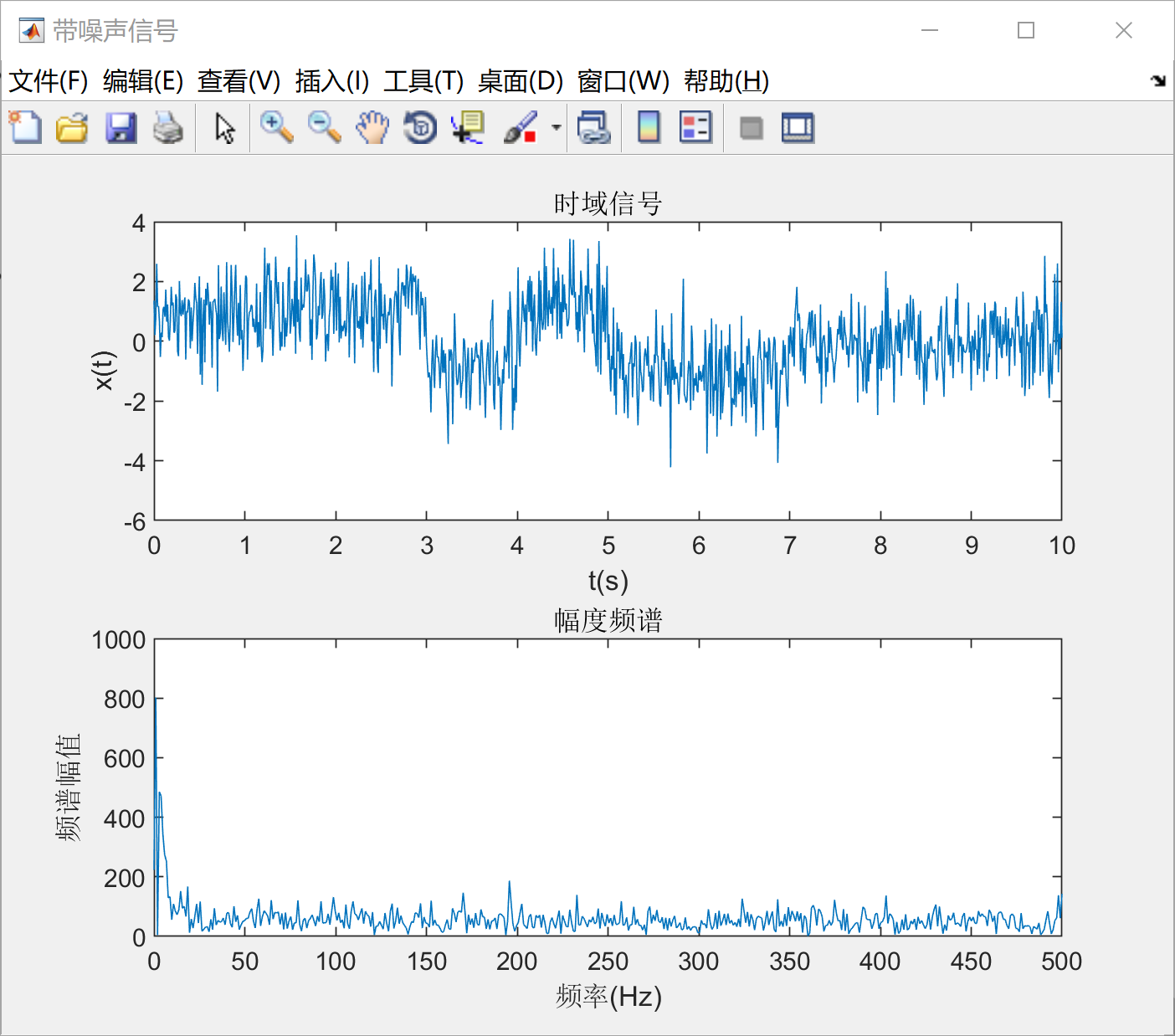LPF的带宽=2Hz
LPF的带宽=2Hz：
LPF=stepfun(f_LPF,0)-stepfun(f_LPF,2);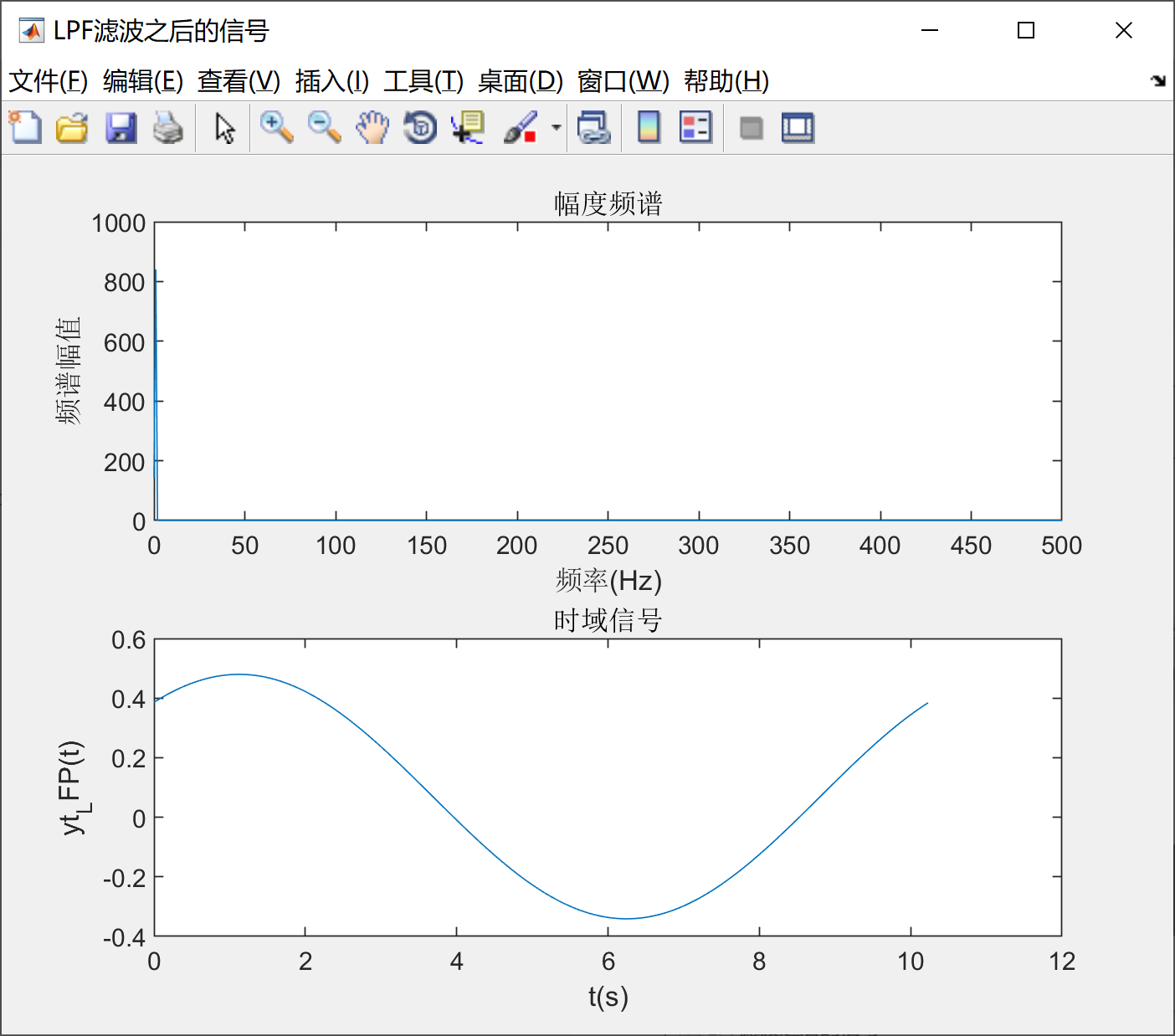LPF的带宽=200Hz：
LPF的带宽=200Hz：
LPF=stepfun(f_LPF,0)-stepfun(f_LPF,200);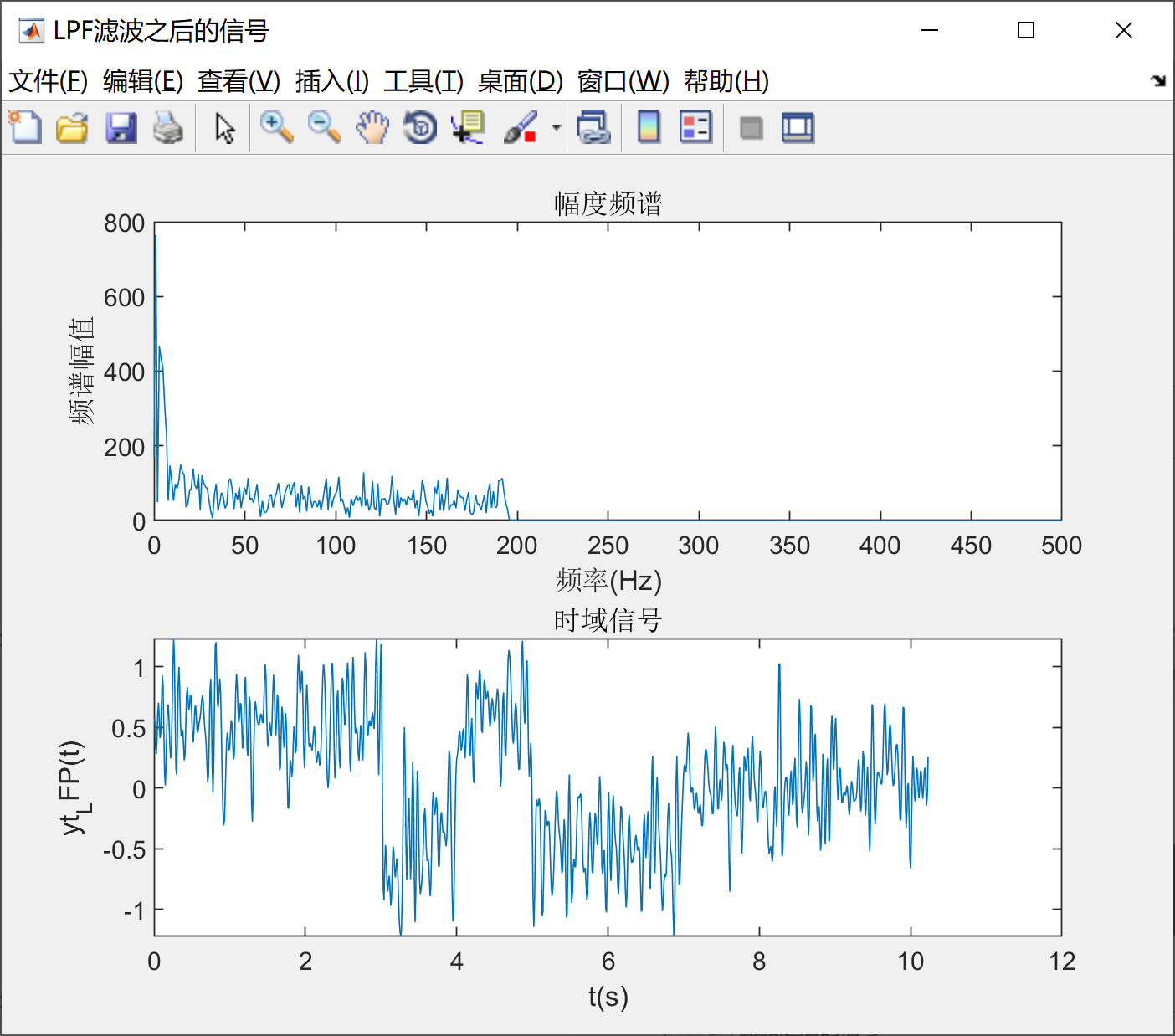展开全文信号处理
• ## 匹配滤波

千次阅读 多人点赞 2020-09-23 20:45:27
匹配滤波（matched filtering）是最佳滤波的一种。当输入信号具有某一特殊波形时，其输出达到最大。在形式上，一个匹配滤波器由以按时间反序排列的输入信号构成。且滤波器的振幅特性与信号的振幅谱一致。因此，对...
    匹配滤波（matched filtering）是最佳滤波的一种。当输入信号具有某一特殊波形时，其输出达到最大。在形式上，一个匹配滤波器由以按时间反序排列的输入信号构成。且滤波器的振幅特性与信号的振幅谱一致。因此，对信号的匹配滤波相当于对信号进行自相关运算。配滤波器是一种非常重要的滤波器，广泛应用与通信、雷达等系统中。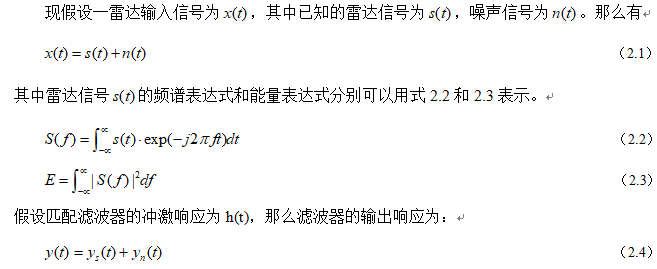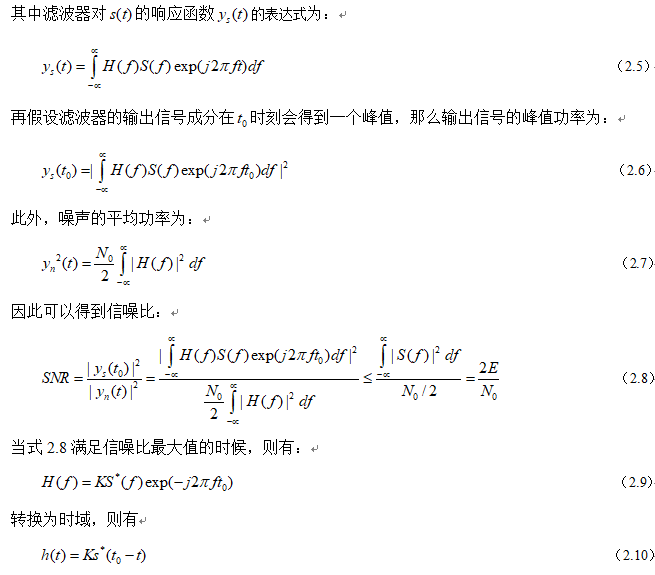从上面的理论推导可以看到，当输出信噪比为最大值的时候，滤波器的传递函数与输入信号的频谱函数满足特定的关系，式2.10就反映了这个关系。满足这种关系的线性滤波器，称之为匹配滤波器。
匹配滤波器的在数学上的运算作用就是对输入信号s(t)做相关运算。在时刻，信号各频率分量同相叠加，得到最大输出值，其输出值的大小只与信号能量有关。
通过上面的分析可知，所谓的最优滤波器，实际上都是在某个准则下的最优。匹配滤波器对应的最优的准则是输出信噪比(SNR)最大。而且还有一个前提条件是在白噪声背景下。 匹配滤波器在很多场合有应用，本课题的雷达信号脉冲压缩技术中匹配滤波器是一个核心功能模块，在MATLAB中仿真，可以得到匹配滤波前后的仿真效果图如图2.1所示。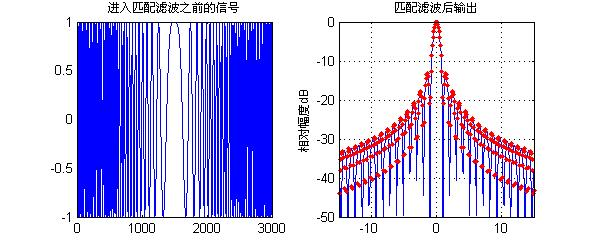图2.1 雷达信号进入匹配滤波器前后对比仿真图
从上面的仿真可以看到，当信号通过匹配滤波器之后，信号的在某一时刻会产生一个最大值，这个最大值就是滤波器的输出值，其胖瓣会产生明显的衰减现象。通过匹配滤波之后的雷达信号，由于对其进行目标的跟踪与搜索。
通过上面的理论分析和仿真可以知道，匹配滤波器具备如下的特征：
一方面，从幅频特性来看，匹配滤波器和输入信号的幅频特性完全一样。这也就是说，在信号越强的频率点，滤波器的放大倍数也越大；在信号越弱的频率点，滤波器的放大倍数也越小。也就是说，匹配滤波器是让信号尽可能通过，而不管噪声的特性。
另外一方面，从相频特性上看，匹配滤波器的相频特性和输入信号正好完全相反。这样，通过匹配滤波器后，信号的相位为0，正好能实现信号时域上的相干叠加。而噪声的相位是随机的，只能实现非相干叠加。这样在时域上保证了输出信噪比的最大。
实际上，在信号与系统的幅频特性与相频特性中，幅频特性更多地表征了频率特性，而相频特性更多地表征了时间特性。匹配滤波器无论是从时域还是从频域，都充分保证了信号尽可能大地通过，噪声尽可能小地通过，因此能获得最大信噪比的输出。
展开全文• matlab匹配滤波代码FPGA通用定点FIR滤波器实现 - 主要特点： 1订单： 并行FIR架构和测试平台设计为可以接受任意数量的抽头，从而可以接受任何滤波器阶数。 由于已完全流水线化，因此增加滤波器的阶数将不会影响最大...
• 匹配滤波器设计代码，有注释，帮助理解匹配滤波器的应用
• 雷达线性调频下的匹配滤波以及脉冲压缩作用的仿真分析，里面包含原理讲解，公式推导，matlab仿真及分析
• matlab匹配滤波代码mexopencv 用于OpenCV库的matlab mex函数的集合和开发套件 该软件包提供了与一百个OpenCV API接口的matlab mex函数。 该包还包含在Matlab的本机数据类型和OpenCV数据类型之间转换的C ++类。 该...
• 包含多种匹配滤波方法的设计，以及QPSK、MFSK、QAM的应用工程。
• matlab匹配滤波代码3DSIFT 3D SIFT的多线程CPU实现，这是3D图像（体积图像）的尺度不变特征变换（SIFT）。 还提供了3D SIFT特征的特征匹配。 读取NIFTI图像的API包含在此程序中。 该程序是用C ++语言编写的，并使用...
• matlab匹配滤波代码平行束CT 参考 不熟悉CT概念和原理的初学者，请阅读第3章。 抽象的 X射线计算机断层扫描（CT）在过去的25年中经历了技术发展的爆炸式增长。 在第二版《计算机断层扫描》问世六年之后，该第三版...
• matlab匹配滤波代码MFLib-匹配的过滤库 包含用于执行匹配过滤检测的源代码。 原则上，该算法非常简单，因为它会在与模板相对应的时间序列中的每个样本上计算a。 但是，以编译语言进行的实际实现是乏味的。 安装 请...
• matlab匹配滤波代码星空 StarryNite是StarryNite细胞谱系软件包的当前Matlab版本，最初是为秀丽隐杆线虫构建的，但已应用于许多模型。 要从源代码运行StarryNite，请将3个源目录的父目录添加到matlab路径，并以......

# 匹配滤波matlab实现matlab 订阅